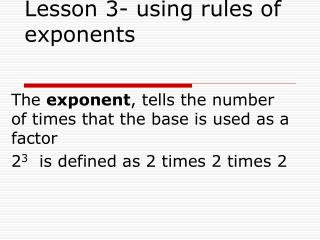Download PresentationLesson 3- using rules of exponents

# Lesson 3- using rules of exponents - PowerPoint PPT PresentationDownload Presentation## Lesson 3- using rules of exponents

- - - - - - - - - - - - - - - - - - - - - - - - - - - E N D - - - - - - - - - - - - - - - - - - - - - - - - - - -
##### Presentation Transcript

1. Lesson 3- using rules of exponents The exponent, tells the number of times that the base is used as a factor 23 is defined as 2 times 2 times 2

2. Definition of negative exponents • X-n = 1/xn • 2-3 = 1/23

3. simplfy • 1/3-2 • 3-3 • -3-2 • 3-3 • 1/5-2 • 5-2 • -5-2 • (-5) -2 • -(-5) -2

4. Product rule for exponents • xm xn = x m+n

5. Power rule for exponents • (xm)n= xmn

6. Scientific notation • Multiplying by a positive power of 10 , moves the decimal point to the right. • Multiplying by a negative power of 10, moves the decimal point to the left

7. Simplifying expressions in scientific notation • (4.2 x 10-7)(.0028) • 2400(1.6 x 10-10) • (.0003 x 10-6)(4000) • (.006 x 1015)(2000 x 104)

8. Lesson 6 finding percent of change • Any fraction or decimal can be written as a percent. • To write a decimal as a percent, multiply by 100(move decimal point 2 places to the left) • To write a fraction as a percent, divide the numerator by the denominator, then multiply by 100

9. examples • Change .12 to a percent • Change 8 to a percent • Change 4/5 to a percent • Change 22/8 to a percent • Change .035 to a percent

10. Percent of change • Percent of change is the increase or decrease given as a percent of the original amount • When the new amount is greater than the original amount, the change is a percent increase • When the new amount is less then the original amount, the change is a percent decrease

11. Percent of change • Percent of change= amount of increase or decrease • original amount

12. practice • Calculate the percent of change and tell whether it is an increase or decrease. • From 120 to 168 • From 6 to 5.1 • From 18 to 72 • From 240 to 60

13. Determining new amount • What is the new amount when 58 is decreased by 70%? • 1. find amount of decrease • 70% of 58= .70x58 = 40.6 • 2. subtract amount of decrease from original amount • 58-40.6= 17.4 • OR • 1. subtract 70% from 100% = 30% • 2. find 30% of 58 = 17.4

14. What is the new amount when 125 is decreased by 15% • 1. .15 x 125=18.75 • 2. 125-18.75= 106.25 • Or • 1. 100%- 15%= 85% • 2. 85% x 125 = 106.25

15. Find new amount when 620 is increased by 350%. • 1. find 350% of 620 = 3.5 x 620 =2170 • 2.add 2170 to 620= 2790 • Or • 1. add 100% to 350%= 450% • 2. find 450% of 620= 4.5 x 620= 2790

16. discount • When an item in a store is on sale, the new price reflects a discount. • The amount of discount is the difference between the old and new price and the percent of discount is a percent of discount

17. When the price of an item increases, the increase is a markup, and the percent of change is a percent increase. • A markup can refer to an increase in the retail price of an item or it can refer to the amount by which the wholesale cost of the item is increased. • Marked up price=original price +markup • =(wholesale price) + markup • Sale price = original price - discount

18. Find sale price • A digital camera costs \$128. It is being offered at a 25% discount. Find the sale price. • Sale price = original price - discount • S = 128 - 25% x 128 • S = 128 -32= \$96 • Or • 100% -25% = 755 • 75% of 128 = 96

19. Finding marked up price • A grocer buys watermelon from a farmer for \$1.34 each and sells them at a 250% markup. What is the cost of the watermelon at the grocer's store. • Marked up price= wholesale price+ markup • = 1.34+ 250% of 1.34 • =1.34 + 3.35 • = 4.69

20. Check for understanding • Explain how to find the new amount when a number is increased by a percent. • Explain the difference between a discount and a markup. • A student states that a negative exponent means the exponential must be in the denominator. Is this correct? Explain.